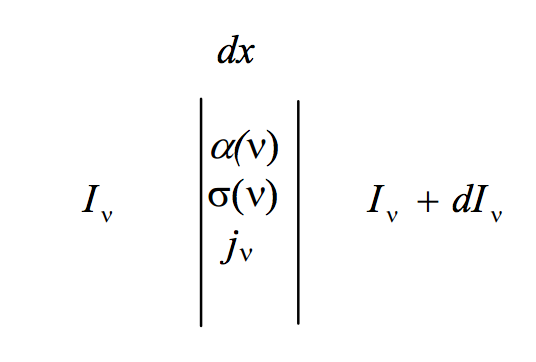$$\require{cancel}$$

# 5.5: The Equation of Transfer

•• Contributed by Jeremy Tatum
• Emeritus Professor (Physics & Astronomy) at University of Victoria

The equation of transfer deals with the transfer of radiation through an atmosphere that is simultaneously absorbing, scattering and emitting.$$\text{FIGURE V.1}$$

Suppose that, between $$x$$ and $$x + dx$$ the absorption coefficient and the scattering coefficient at frequency $$\nu$$ are $$\alpha (\nu)$$ and $$\sigma (\nu)$$, and the emission coefficient per unit frequency interval is $$j_{\nu} d\nu$$. In this interval, suppose that the specific intensity per unit frequency interval increases from $$I_{\nu}$$ to $$I_{\nu} + dI_{\nu}$$ ($$dI_{\nu}$$ might be positive or negative). The specific intensity will be reduced by absorption and scattering and increased by emission. Thus:

$dI_{\nu} = - [I_{\nu} \alpha (\nu) + I_{\nu} \sigma (\nu) - j_{\nu} (\nu) ] dx. \label{5.5.1} \tag{5.5.1}$

This is one form - the most basic form - of the equation of transfer. Notice that $$\alpha$$ and $$\sigma$$ do not have a subscript.